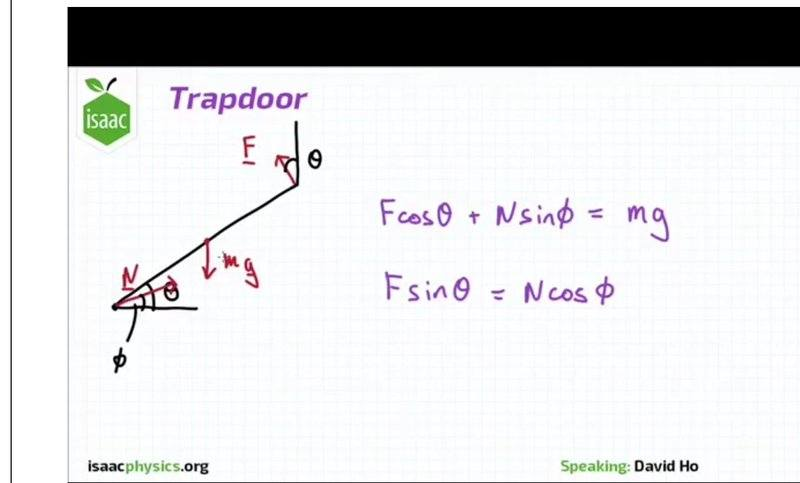# Find Value of tan φ of Reaction Force | Isaac Physics

• ephphatha16

#### ephphatha161.
Find the value of tan φ to 2 sf where φ is angle made by line of action of reaction force at hinge above horizontal.
https://isaacphysics.org/questions/trapdoor_num
Part B Angle of reaction force

## Homework Equations

• Mass of uniform trapdoor: m=4.0kgm=4.0kg

• The trapdoor is held open making an angle θ=60∘θ=60∘ to the horizontal.
Information assumed
- Magnitude of the gravitational field strength, g=9.81ms−2

## The Attempt at a Solution

[/B]Don't know how to move on with this simultaneous equation

Fcosθ + Nsinθ=mg
Fsinθ = Ncosθ

#### Attachments

Last edited:
Fcosθ + Nsinθ=mg
Fsinθ = Ncosθ
Shouldn't some of those angles be φ?
You will also need to consider torque balance.

•ephphatha16
Shouldn't some of those angles be φ?
You will also need to consider torque balance.
Yeah true

Fcos θ + Nsin φ = mg
F sin θ = N cos φ

But I don't know what values to plug in ... is the first equation about vertical component and the second equation about horizontal component?

I don't know what values to plug in
φ will not depend on m or g. You are given a value for θ.
is the first equation about vertical component and the second equation about horizontal component?
Yes, but you have three unknowns, F/mg, N/mg and φ. You need a torque balance equation.

•ephphatha16